• +91 9971497814
• info@interviewmaterial.com

# RD Chapter 28- Introduction to 3D coordinate geometry Ex-28.3 Interview Questions Answers

### Related Subjects

Question 1 : The vertices of the triangle are A(5, 4, 6), B(1, -1, 3) and C(4, 3, 2). The internal bisector of angle A meets BC at D. Find the coordinates of D and the length AD.

Given:

The vertices of the triangle are A (5, 4, 6), B (1, -1, 3) and C(4, 3, 2).

By using the formulas let us find the coordinates of D and thelength of AD

The distance between any two points (a, b, c) and (m, n, o)is given by,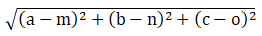The section formula is given as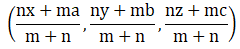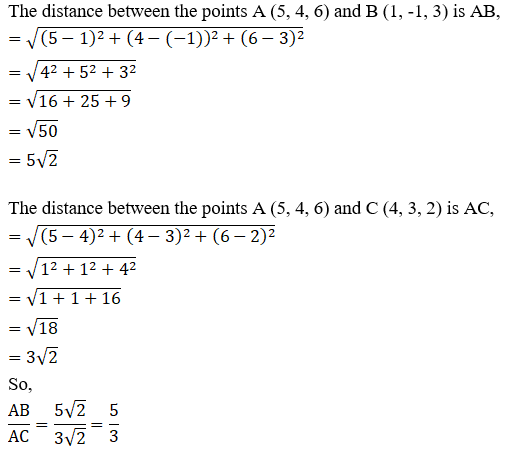AB : AC = 5:3

BD: DC = 5:3

So, m = 5 and n = 3

B(1, -1, 3) and C(4, 3, 2)

Coordinates of D using section formula: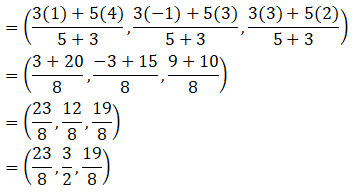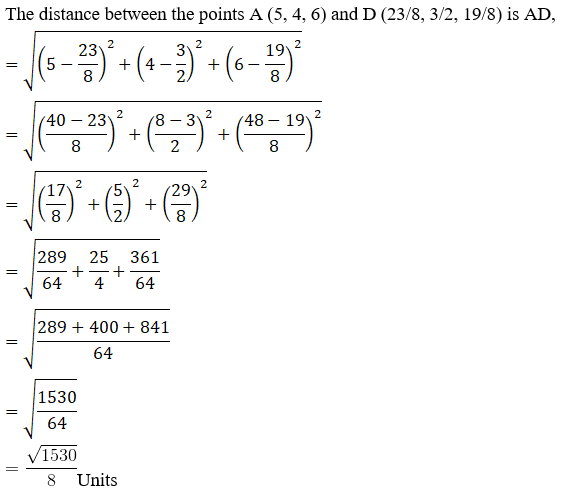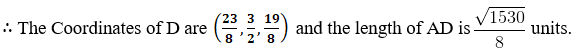Question 2 : A point C with z-coordinate 8 lies on the line segment joining the points A(2, -3, 4) and B(8, 0, 10). Find the coordinates.

Given:
The points A (2, -3, 4) and B (8, 0, 10)
By using the section formula,Let Point C(x, y, 8), and C divides AB in ratio k: 1
So, m = k and n = 1
A(2, -3, 4) and B(8, 0, 10)
Coordinates of C are: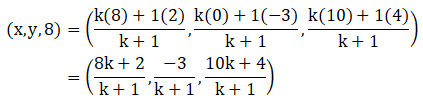On comparing we get,
[10k + 4] / [k + 1] = 8
10k + 4 = 8(k + 1)
10k + 4 = 8k + 8
10k – 8k = 8 – 4
2k = 4
k = 4/2
= 2
Here C divides AB in ratio 2:1
x = [8k + 2] / [k + 1]
= [8(2) + 2] / [2 + 1]
= [16 + 2] / 
= 18/3
= 6
y = -3 / [k + 1]
= -3 / [2 + 1]
= -3 / 3
= -1
∴ The Coordinates of C are (6, -1, 8).

Question 3 : Show that the three points A(2, 3, 4), B(-1, 2, -3) and C(-4, 1, -10) are collinear and find the ratio in which C divides AB.

Given:
The points A (2, 3, 4), B (-1, 2, -3) and C (-4, 1, -10)
By using the section formula,Let C divides AB in ratio k: 1
Three points are collinear if the value of k is the same for x, y and z coordinates.
So, m = k and n = 1
A(2, 3, 4), B(-1, 2, -3) and C(-4, 1, -10)
Coordinates of C are: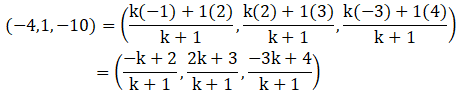On comparing we get,
[-k + 2] / [k + 1] = -4
-k + 2 = -4(k + 1)
-k + 2 = -4k – 4
4k – k = – 2 – 4
3k = -6
k = -6/3
= -2
[2k + 3] / [k + 1] = 1
2k + 3 = k + 1
2k – k = 1 – 3
k = – 2
[-3k + 4] / [k + 1] = -10
-3k + 4 = -10(k + 1)
-3k + 4 = -10k – 10
-3k + 10k = -10 – 4
7k = -14
k = -14/7
= -2
The value of k is the same in all three cases.
So, A, B and C are collinear [as k = -2]
∴We can say that, C divides AB externally in ratio 2:1

Question 4 : Find the ratio in which the line joining (2, 4, 5) and (3, 5, 4) is divided by the yz-plane.

Given:
The points (2, 4, 5) and (3, 5, 4)
By using the section formula,We know X coordinate is always 0 on yz-plane
So, let Point C(0, y, z), and let C divide AB in ratio k: 1
Then, m = k and n = 1
A(2, 4, 5) and B(3, 5, 4)
The coordinates of C are: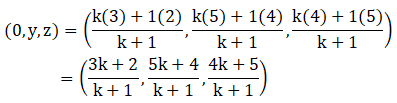On comparing we get,
[3k + 2] / [k + 1] = 0
3k + 2 = 0(k + 1)
3k + 2 = 0
3k = – 2
k = -2/3
∴We can say that, C divides AB externally in ratio 2: 3

Question 5 : Find the ratio in which the line segment joining the points (2, -1, 3) and (-1, 2, 1) is divided by the plane x + y + z = 5.

Given:
The points(2, -1, 3) and (-1, 2, 1)
By using the section formula,Let C(x, y, z) be any point on the given plane and C divides AB in ratio k: 1
Then, m = k and n = 1
A(2, -1, 3) and B(-1, 2, 1)
Coordinates of C are: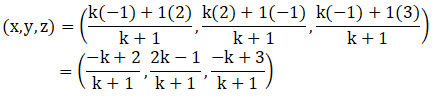On comparing we get,

[-k + 2] / [k + 1] = x

[2k – 1] / [k + 1] = y

[-k + 3] / [k + 1] = z

We know that x + y + z = 5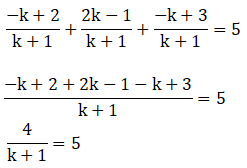5(k + 1) = 4

5k + 5 = 4

5k = 4 – 5

5k = – 1

k = -1/5

We can saythat, the plane divides AB externally in the ratio 1:5

Question 6 : If the points A(3, 2, -4), B(9, 8, -10) and C(5, 4, -6) are collinear, find the ratio in which C divided AB.

Given:

The points A (3, 2,-4), B (9, 8, -10) and C (5, 4, -6)

By using the sectionformula,Let C divides AB inratio k: 1

Three points arecollinear if the value of k is the same for x, y and z coordinates.

Then, m = k and n = 1

A(3, 2, -4), B(9, 8,-10) and C(5, 4, -6)

Coordinates ofC are: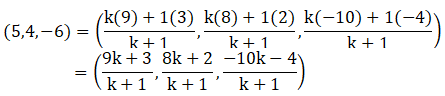On comparing we get,

[9k +3] / [k + 1] = 5

9k + 3 = 5(k + 1)

9k + 3 = 5k + 5

9k – 5k = 5 – 3

4k = 2

k = 2/4

= ½

[8k +2] / [k + 1] = 4

8k + 2 = 4(k + 1)

8k + 2 = 4k + 4

8k – 4k = 4 – 2

4k = 2

k = 2/4

= ½

[-10k– 4] / [k + 1] = -6

-10k – 4 = -6(k + 1)

-10k – 4 = -6k – 6

-10k + 6k = 4 – 6

-4k = -2

k = -2/-4

= ½

The value of k is thesame in all three cases.

So, A, B and Care collinear [as, k = ½]

We can say that, Cdivides AB externally in ratio 1:2

Question 7 : The mid-points of the sides of a triangle ABC are given by (-2, 3, 5), (4, -1, 7) and (6, 5, 3). Find the coordinates of A, B and C.

Given:

The mid-points of thesides of a triangle ABC is given as (-2, 3, 5), (4, -1, 7) and (6, 5, 3).

By using the sectionformula,We know the mid-pointdivides side in the ratio of 1:1.

The coordinates of Cis given by,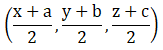P(-2, 3, 5) ismid-point of A(x1, y1, z1) and B(x2,y2, z2)

Then,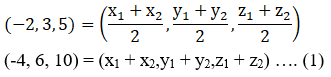Q(4, -1, 7) ismid-point of B(x2, y2, z2) and C(x3,y3, z3)

Then,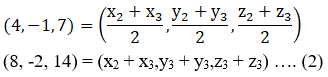R(6, 5, 3) ismid-point of A(x1, y1, z1) and C(x3,y3, z3)

Then,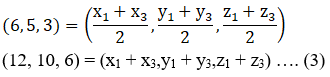Now solving for ‘x’terms

x1 + x2 =-4……………………(4)

x2 + x3 =8………………………(5)

x1 + x3 =12……………………(6)

x1 + x2 +x2 + x3 + x1 + x3 =8 + 12 – 4

2x1 +2x2 + 2x3 = 16

2(x1 +x2 + x3) = 16

x1 + x2 +x3 = 8………………………(7)

Now, subtract equation(4), (5) and (6) from equation (7) separately:

x1 + x2 +x3 – x1 – x2 = 8 – (-4)

x3 =12

x1 + x2 +x3 – x2 – x3 = 8 – 8

x1 = 0

x1 + x2 +x3 – x1 – x3 = 8 – 12

x2 =-4

Now solving for ‘y’terms

y1 + y2 =6……………………(8)

y2 + y3 =-2……………………(9)

y1 + y3 =10……………………(10)

By adding equation(8), (9) and (10) we get,

y1 + y2 +y2 + y3 + y1 + y3 =10 + 6 – 2

2y1 +2y2 + 2y3 = 14

2(y1 +y2 + y3) = 14

y1 + y2 +y3 = 7………………………(11)

Now, subtract equation(8), (9) and (10) from equation (11) separately:

y1 + y2 +y3 – y1 – y2 = 7 – 6

y3 = 1

y1 + y2 +y3 – y2 – y3 = 7 – (-2)

y1 = 9

y1 + y2 +y3 – y1 – y3 = 7 – 10

y2 =-3

Now solving for ‘z’terms

z1 + z2 =10……………………(12)

z2 + z3 =14……………………(13)

z1 + z3 =6……………………(14)

By adding equation(12), (13) and (14) we get,

z1 + z2 +z2 + z3 + z1 + z3 =6 + 14 + 10

2z1 +2z2 + 2z3 = 30

2(z1 +z2 + z3) = 30

z1 + z2 +z3 = 15………………………(15)

Now, subtract equation(8), (9) and (10) from equation (11) separately:

z1 + z2 +z3 – z1 – z2 = 15 – 10

z3 = 5

z1 + z2 +z3 – z2 – z3 = 15 – 14

z1 = 1

z1 + z2 +z3 – z1 – z3 = 15 – 6

z2 = 9

Thevertices of sidesof a triangle ABC are A(0, 9, 1) B(-4,-3, 9) and C(12, 1, 5).

Question 8 : A(1, 2, 3), B(0, 4, 1), C(-1, -1, -3) are the vertices of a triangle ABC. Find the point in which the bisector of the angle ∠BAC meets BC.

Given:
The vertices of a triangle are A (1, 2, 3), B (0, 4, 1), C (-1, -1, -3)
By using the distance formula,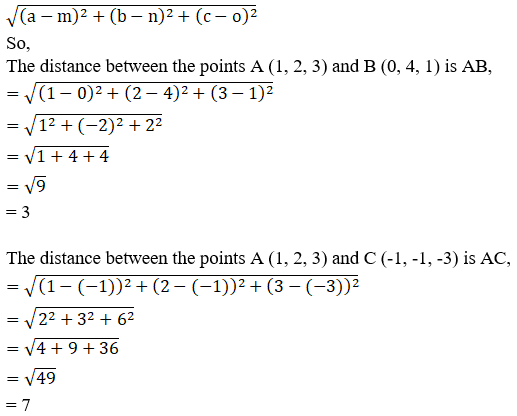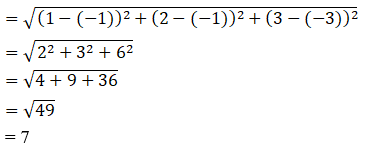So, AB/AC = 3/7

AB: AC = 3:7

BD: DC = 3:7

Then, m = 3 and n = 7

B(0, 4, 1) and C(-1,-1, -3)

Coordinates of D byusing section formula is given as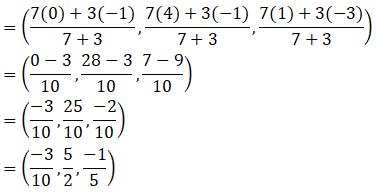The coordinates of Dare (-3/10, 5/2, -1/5).

Todays Deals### RD Chapter 28- Introduction to 3D coordinate geometry Ex-28.3 Contributorskrishan

Name:
Email:

# Latest News# 9000 interview questions in different categories